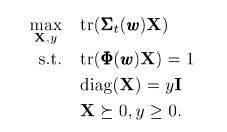# Maximizing the objective function with the complex variable

Hello guys, I’m new with CVX and have some optimization problems. I am trying to optimize the SDP problem as following:I made some changes to the variable name and the code as following:

cvx_begin
variable M_s(NM_t,NM_t) complex semidefinite
variable t
maximize trace(real(X_wM_s))
subject to
trace(R_DL
M_s)==1;
for tt=1:NM_t
M_s(tt,tt)==t;
end
M_s==hermitian_semidefinite(N
M_t);
t>=0;
cvx_end

I’m not sure if the function ‘real’ can affect the output. Is my matlab cvx code right?

You are allowed to use real wherever you want in CVX. Even if you don’t theoretically need “real” if an expression would be real in exact arithmetic, sometimes it is necessary in order to deal with a roundoff level imaginary term.

I don’t see anything obviously wrong with your code, but ultimately, you know better than I do what the original problem is, and the properties of its input data.

Have you run your cide? Did you get a solution?

These code is part of my simulation, I’m worried about whether this operation ‘real’ will lose some information because I can’t get the result which I want. (although this SDP problem was solved)

Thank you for helping me to make sure there is nothing wrong with my code. So I will find mistakes from somewhere else.dear Mark, can you teach me how to solve the quasi-convex problem with dichotomy?
I can understand its ideas, but how should I use the function ‘find’?

I have no idea what you’re talking about with “dichotomy” and “find”.

If you have a quasi-convex function which you want to solve for which the feasibility problems in the bisection algorithm can be represented in CVX, you can find a bisection algorithm description in section 4.2.5 “Quasiconvex optimization” of Boyd and Vandenberghe “Convex Optimization” https://web.stanford.edu/~boyd/cvxbook/bv which you can implement in a MATLAB for loop inside of which is a CVX convex feasibility problem.

If you are referring to some MATLAB user-contributed “dichotomy” function, whatever it might do, the CVX Forum is not the right place to get help with it.

I’m sorry for my inappropriate expression. What I want to know is how to implement the algorithm which you said in a MATLAB… Should I use the ‘cvx_status’ to judge whether the problem is feasibility? Could you show me some examples?

Here is an example of performing bisection with CVX https://github.com/cvxr/CVX/blob/master/examples/filter_design/fir_lin_phase_lowpass_min_trans.m .

Thank you very much!!